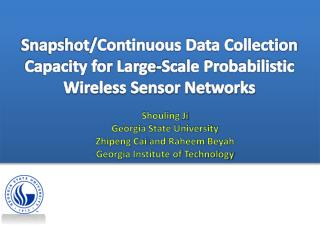DownloadDownload PresentationShouling Ji Georgia State University Zhipeng Cai and Raheem Beyah

# Shouling Ji Georgia State University Zhipeng Cai and Raheem Beyah

Download Presentation## Shouling Ji Georgia State University Zhipeng Cai and Raheem Beyah

- - - - - - - - - - - - - - - - - - - - - - - - - - - E N D - - - - - - - - - - - - - - - - - - - - - - - - - - -
##### Presentation Transcript

1. Snapshot/Continuous Data Collection Capacity for Large-Scale Probabilistic Wireless Sensor Networks ShoulingJi Georgia State University ZhipengCai and RaheemBeyah Georgia Institute of Technology

2. OUTLINE 1 Introduction Network Model 2 Network Partition 3 Snapshot Data Collection 4 5 Continuous Data Collection 6 Simulation 7 Conclusion

3. Introduction

4. Introduction • Capacity analysis in WSNs • Why? • Unicast, Multicast, and Broadcast capacity • Bits/Meter/Second • Data Collection Capacity • Snapshot Data Collection Capacity • Continuous Data Collection Capacity

5. Introduction • Deterministic network model • Transitional region phenomenon • Probabilistic network model • Contributions • A Cell-based Multi-Path Scheduling (CMPS) algorithm for snapshot data collection in probabilistic WSNs • A Zone-based Pipeline Scheduling (ZPS) algorithm for continuous data collection in probabilistic WSNs

6. Network Model

7. Network Model • n sensor nodes, , • i.i.d. deployed in a square area • The sink is located at the top-right corner of the square • Single-radio single-channel • Success probability of a link

8. Network Model • The number of transmission times satisfies the geometric distribution with parameter • Promising transmission threshold probability • A modified time slot • Data collection capacity

9. Network Partition

10. Network Partition • Cell-based network partition • The expected number of nodes in each cell . (Lemma 1) • It is almost surely that no cell is empty. (Lemma 2) • It is almost surely that no cell contains more than nodes. (Lemma 3)

11. Network Partition • Zone-based network partition • Compatible Transmission Cell Set (CTCS) • Let then the set is a CTCS. (Theorem 1)

12. Snapshot Data Collection

13. Snapshot Data Collection • Data collection tree • Super node, super time slot

14. Snapshot Data Collection • Cell-based Multi-Path Scheduling (CMPS) • Phase I: Inner-Tree Scheduling. Schedule CTCSs orderly. • Phase II: Schedule .

15. Snapshot Data Collection • Analysis • It takes CMPS super time slots to finish Phase I. (Lemma 6) • Let be the number of super data packets transmitted by super node through the data collection process. Then, for , (Lemma 7) • Let be the number of super data packets at waiting for transmission at the beginning of Phase II and , then (Lemma 8)

16. Snapshot Data Collection • Analysis • The achievable data collection capacity of CMPS is in the worst cast and in the average case. In both cases, CMPS is order-optimal. (Theorem 2)

17. Continuous Data Collection

18. Continuous Data Collection • Continuous Data Collection • Compressive Data Gathering + pipeline • Zone-based Pipeline Scheduling (ZPS) algorithm • Inter-Segment Pipeline Scheduling. • Intra-Segment Scheduling.

19. Continuous Data Collection • Analysis • To collection N continuous snapshots, the achievable network capacity of ZPS is in the worst case, and in the average case. (Theorem 3)

20. Simulation

21. Simulation • Network Setting • Parameters  • CMPS • PS , MPS  • ZPS • PSP (PS + pipeline) [PS], CDGP (CDG + pipeline) , PSA 

22. Simulation • Performance of CMPS

23. Simulation • Performance of ZPS

24. Simulation • Performance of CMPS and ZPS in deterministic WSNs

25. Conclusion • We proposed a snapshot data collection algorithm CMPS for probabilistic WSNs, whose capacity is proven to be order-optimal • We proposed a continuous data collection algorithm ZPS for probabilistic WSNs, and analyzed its performance • Extensive simulations validated that the proposed algorithms can accelerate the data collection process

26. Snapshot/Continuous Data Collection Capacity for Large-Scale Probabilistic Wireless Sensor Networks ShoulingJi and ZhipengCai Georgia State University RaheemBeyah Georgia Institute of Technology Thank you!